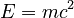# Conservation law facts for kids

Kids Encyclopedia Facts

A conservation law is a statement used in Physics that says that the amount of something does not change in time. That thing could be as simple as mass or charge, or something that has to be calculated, like energy, or angular momentum.

For example, the "law of conservation of mass" is the conservation law that says that the amount of mass is always conserved, even if it is changed into another form. This means that if the mass of the universe could be measured right now, its mass would be known tomorrow because it will not change.

## History

For a long time, people thought that these laws were true for the amount of mass and energy in the universe. Later on, Albert Einstein said that they were not completely true. He said that mass could change into energy (or the other way around). If this happened, it would be against the conservation laws because if mass was changed into energy, the total amount of mass goes down, and the total amount of energy goes up.

Einstein said that conservation laws could still be used if all the mass and all the energy were combined. He said that even though the mass changes or the energy changes, the sum when they are added together does not change. So now there is just one conservation law for mass and energy together.

### Problems

Of course, mass is measured in kilograms, and energy is measured in joules. They cannot be added together directly, but Einstein found a way to add them together. He created the equation$E=mc^2$. This equation means is that before adding the amount of mass to the amount of energy, the mass must be multiplied by the speed of light and then by the speed of light again.

## Purpose

Some of the things that are thought to be conserved are:

Conservation laws are helpful for people when they do problems in Physics. This is because if they know that a thing is conserved, it gives them more mathematical information about the thing they are doing the problem about.

Emmy Noether showed that conservation laws can be said to arise from symmetries in the laws of physics. This theorem, called Noether's theorem gives physicists an extremely powerful tool to try and solve complicated problems.

For example:

• Since there is no absolute position, only relative position, we can get that the total momentum of a closed system is conserved.
• Since there is no absolute time, only relative time, we can get that the total energy of a closed system is conserved.
• Since there is no absolute orientation or preferred direction in space, only relative orientation, we can get that the total angular momentum of a closed system is conserved.
• There are more sophisticated symmetries, like local gauge invariance which lead to the conservation of charge.

## Types of Conservation laws

Conservation laws can come in two types, global, or local.

### Global conservation

A global conservation law just says that the total amount of something in the universe does not change in time.

### Local conservation

A local conservation law says a little bit more than that. It says that if the amount of something changed in one place, it's because it moved in to or out of that place, and we can measure that movement.Conservation law Facts for Kids. Kiddle Encyclopedia.